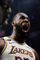cancel
Showing results for
Did you mean:Helper I

## Average of column filtered by month

Hello all,

I am working with a data set that has three columns (designed below) - 1) Order date - 2) "Was the order included" - 3) Site Name.

 Order Date Was the order included? Site Name 09/02/2020 Y Danville 10/05/2020 N Clinch 10/22/2020 N Dallas North 11/22/2020 Y Dallas North 11/09/2020 Y Dallas North

What I'm trying to do is use the "Was the order included" column to get an average score of the number of orders that were included (ie "Y" for that row) filtered out for each month.
For example: If for the month of October -- Clinch had a total of 50 orders and 45 of them were included (ie 45 with "Y" and 5 with "N") then the average for that month would show as 90%

How can I set this formula up for my report?

Thanks

M.R

1 ACCEPTED SOLUTIONCommunity Support

Hi, @mrizvi

Depending on your needs, the following Measure is recommended

Measure =

var _month = MONTH(SELECTEDVALUE('Table'[Order Date]))

var a =

CALCULATE(

COUNTROWS('Table'),

FILTER(

ALL('Table'),

'Table'[Was the order included?] = "Y" &&

MONTH('Table'[Order Date]) = _month

)

)

var b =

CALCULATE(

COUNTROWS('Table'),

FILTER(

ALL('Table'),

MONTH('Table'[Order Date]) = _month

)

)

return

a / b

The result is as follows:Here is the sample file that you can refer:

Best Regards,

If this post helps then please consider Accept it as the solution to help the other members find it more quickly.

2 REPLIES 2Community Support

Hi, @mrizvi

Depending on your needs, the following Measure is recommended

Measure =

var _month = MONTH(SELECTEDVALUE('Table'[Order Date]))

var a =

CALCULATE(

COUNTROWS('Table'),

FILTER(

ALL('Table'),

'Table'[Was the order included?] = "Y" &&

MONTH('Table'[Order Date]) = _month

)

)

var b =

CALCULATE(

COUNTROWS('Table'),

FILTER(

ALL('Table'),

MONTH('Table'[Order Date]) = _month

)

)

return

a / b

The result is as follows:Here is the sample file that you can refer:

Best Regards,

If this post helps then please consider Accept it as the solution to help the other members find it more quickly.Super User

@mrizvi , Create a measure like this and try

divide(calculate(countrows(Table), filter(Table,Table[Was the order included] ="Y")),countrows(Table))

!! Microsoft Fabric !!
Microsoft Power BI Learning Resources, 2023 !!
Learn Power BI - Full Course with Dec-2022, with Window, Index, Offset, 100+ Topics !!
Did I answer your question? Mark my post as a solution! Appreciate your Kudos !! Proud to be a Super User! !!